## Section 6.6: Vectors

### Learning Outcomes

• View vectors geometrically.
• Find magnitude and direction.
• Perform vector addition and scalar multiplication.
• Find the component form of a vector.
• Find the unit vector in the direction of $\boldsymbol{v}$ .
• Perform operations with vectors in terms of $\boldsymbol{i}$ and $\boldsymbol{j}$ .
• Find the magnitude and direction of the resultant vector.
• Find the dot product of two vectors.

An airplane is flying at an airspeed of 200 miles per hour headed on a SE bearing of 140°. A north wind (from north to south) is blowing at 16.2 miles per hour, as shown in Figure 1. What are the ground speed and actual bearing of the plane?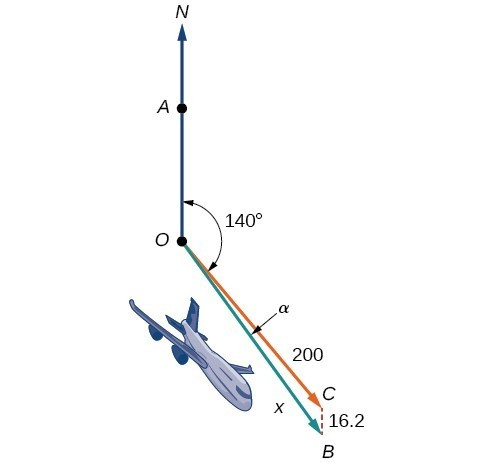Figure 1

Ground speed refers to the speed of a plane relative to the ground. Airspeed refers to the speed a plane can travel relative to its surrounding air mass. These two quantities are not the same because of the effect of wind. In an earlier section, we used triangles to solve a similar problem involving the movement of boats. Later in this section, we will find the airplane’s groundspeed and bearing, while investigating another approach to problems of this type. First, however, let’s examine the basics of vectors.

## A Geometric View of Vectors

A vector is a specific quantity drawn as a line segment with an arrowhead at one end. It has an initial point, where it begins, and a terminal point, where it ends. A vector is defined by its magnitude, or the length of the line, and its direction, indicated by an arrowhead at the terminal point. Thus, a vector is a directed line segment. There are various symbols that distinguish vectors from other quantities:

• Lower case, boldfaced type, with or without an arrow on top such as $\boldsymbol{v,u,w,\stackrel{\to }{v},\stackrel{\to }{u},\stackrel{\to }{w}}$.
• Given initial point $P$ and terminal point $Q$, a vector can be represented as $\stackrel{\to }{PQ}$. The arrowhead on top is what indicates that it is not just a line, but a directed line segment.
• Given an initial point of $\left(0,0\right)$ and terminal point $\left(a,b\right)$, a vector may be represented as $\langle a,b\rangle$.

This last symbol $\langle a,b\rangle$ has special significance. It is called the standard position. The position vector has an initial point $\left(0,0\right)$ and a terminal point $\langle a,b\rangle$. To change any vector into the position vector, we think about the change in the x-coordinates and the change in the y-coordinates. Thus, if the initial point of a vector $\stackrel{\to }{CD}$ is $C\left({x}_{1},{y}_{1}\right)$ and the terminal point is $D\left({x}_{2},{y}_{2}\right)$, then the position vector is found by calculating

\begin{align}\stackrel{\to }{AB}&=\langle {x}_{2}-{x}_{1},{y}_{2}-{y}_{1}\rangle \\ &=\langle a,b\rangle \end{align}

In Figure 2, we see the original vector $\stackrel{\to }{CD}$ and the position vector $\stackrel{\to }{AB}$.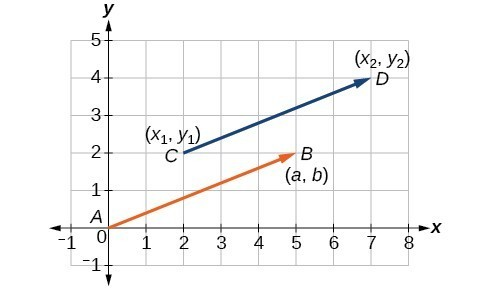Figure 2

### A General Note: Properties of Vectors

A vector is a directed line segment with an initial point and a terminal point. Vectors are identified by magnitude, or the length of the line, and direction, represented by the arrowhead pointing toward the terminal point. The position vector has an initial point at $\left(0,0\right)$ and is identified by its terminal point $\langle a,b\rangle$.

### Example 1: Find the Position Vector

Consider the vector whose initial point is $P\left(2,3\right)$ and terminal point is $Q\left(6,4\right)$. Find the position vector.

### Example 2: Drawing a Vector with the Given Criteria and Its Equivalent Position Vector

Find the position vector given that vector v has an initial point at $\left(-3,2\right)$ and a terminal point at $\left(4,5\right)$, then graph both vectors in the same plane.

### Try It

Draw a vector $\boldsymbol{v}$ that connects from the origin to the point $\left(3,5\right)$.

## Finding Magnitude and Direction

To work with a vector, we need to be able to find its magnitude and its direction. We find its magnitude using the Pythagorean Theorem or the distance formula, and we find its direction using the inverse tangent function.

### A General Note: Magnitude and Direction of a Vector

Given a position vector $\boldsymbol{v}$ $=\langle a,b\rangle$, the magnitude is found by $|\boldsymbol{v}|=\sqrt{{a}^{2}+{b}^{2}}$. The direction is equal to the angle formed with the x-axis, or with the y-axis, depending on the application. For a position vector, the direction is found by $\tan \theta =\left(\frac{b}{a}\right)\Rightarrow \theta ={\tan }^{-1}\left(\frac{b}{a}\right)$, as illustrated in Figure 5.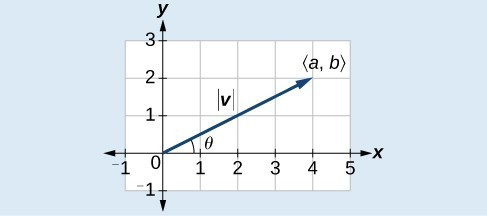Figure 5

Two vectors v and u are considered equal if they have the same magnitude and the same direction. Additionally, if both vectors have the same position vector, they are equal.

### Example 3: Finding the Magnitude and Direction of a Vector

Find the magnitude and direction of the vector with initial point $P\left(-8,1\right)$ and terminal point $Q\left(-2,-5\right)$. Draw the vector.

### Example 4: Showing That Two Vectors Are Equal

Show that vector v with initial point at $\left(5,-3\right)$ and terminal point at $\left(-1,2\right)$ is equal to vector u with initial point at $\left(-1,-3\right)$ and terminal point at $\left(-7,2\right)$. Draw the position vector on the same grid as v and u. Next, find the magnitude and direction of each vector.

## Performing Vector Addition and Scalar Multiplication

Now that we understand the properties of vectors, we can perform operations involving them. While it is convenient to think of the vector $\boldsymbol{u}$ $=\langle x,y\rangle$ as an arrow or directed line segment from the origin to the point $\left(x,y\right)$, vectors can be situated anywhere in the plane. The sum of two vectors u and v, or vector addition, produces a third vector u+ v, the resultant vector.

To find u + v, we first draw the vector u, and from the terminal end of u, we drawn the vector v. In other words, we have the initial point of v meet the terminal end of u. This position corresponds to the notion that we move along the first vector and then, from its terminal point, we move along the second vector. The sum u + v is the resultant vector because it results from addition or subtraction of two vectors. The resultant vector travels directly from the beginning of u to the end of v in a straight path, as shown in Figure 8.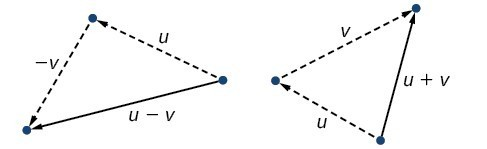Figure 8

Vector subtraction is similar to vector addition. To find uv, view it as u + (−v). Adding −v is reversing direction of v and adding it to the end of u. The new vector begins at the start of u and stops at the end point of −v. See Figure 9 for a visual that compares vector addition and vector subtraction using parallelograms.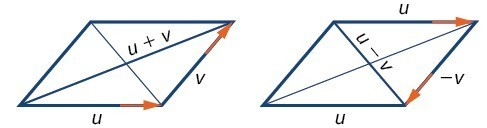Figure 9

### Example 5: Adding and Subtracting Vectors

Given $\boldsymbol{u}$ $=\langle 3,-2\rangle$ and $\boldsymbol{v}$ $=\langle -1,4\rangle$, find two new vectors u + v, and uv.

## Multiplying By a Scalar

While adding and subtracting vectors gives us a new vector with a different magnitude and direction, the process of multiplying a vector by a scalar, a constant, changes only the magnitude of the vector or the length of the line. Scalar multiplication has no effect on the direction unless the scalar is negative, in which case the direction of the resulting vector is opposite the direction of the original vector.

### A General Note: Scalar Multiplication

Scalar multiplication involves the product of a vector and a scalar. Each component of the vector is multiplied by the scalar. Thus, to multiply $\boldsymbol{v}$ $=\langle a,b\rangle$ by $k$ , we have

$k\boldsymbol{v}=\langle ka,kb\rangle$

Only the magnitude changes, unless $k$ is negative, and then the vector reverses direction.

### Example 6: Performing Scalar Multiplication

Given vector $\boldsymbol{v}$ $=\langle 3,1\rangle$, find 3v, $\frac{1}{2}$ v, and −v.

### Try It

Find the scalar multiple $3\boldsymbol{u}$ given $\boldsymbol{u}$ $=\langle 5,4\rangle$.

### Example 7: Using Vector Addition and Scalar Multiplication to Find a New Vector

Given $\boldsymbol{u}=\langle 3,-2\rangle$ and $\boldsymbol{v}=\langle -1,4\rangle$, find a new vector w = 3u + 2v.

## Finding Component Form

In some applications involving vectors, it is helpful for us to be able to break a vector down into its components. Vectors are comprised of two components: the horizontal component is the $x$ direction, and the vertical component is the $y$ direction. For example, we can see in the graph in Figure 12 that the position vector $\langle 2,3\rangle$ comes from adding the vectors v1 and v2. We have v1 with initial point $\left(0,0\right)$ and terminal point $\left(2,0\right)$.

\begin{align}{\boldsymbol{v}}_{1}&=\langle 2 - 0,0 - 0\rangle \\ &=\langle 2,0\rangle \end{align}

We also have v2 with initial point $\left(0,0\right)$ and terminal point $\left(0,3\right)$.

\begin{align}{\boldsymbol{v}}_{2}&=\langle 0 - 0,3 - 0\rangle \\ &=\langle 0,3\rangle \end{align}

Therefore, the position vector is

\begin{align}\boldsymbol{v}&=\langle 2+0,3+0\rangle \\ &=\langle 2,3\rangle \end{align}

Using the Pythagorean Theorem, the magnitude of v1 is 2, and the magnitude of v2 is 3. To find the magnitude of v, use the formula with the position vector.

\begin{align}|\boldsymbol{v}|&=\sqrt{|{\boldsymbol{v}}_{1}{|}^{2}+|{\boldsymbol{v}}_{2}{|}^{2}} \\ &=\sqrt{{2}^{2}+{3}^{2}} \\ &=\sqrt{13} \end{align}

The magnitude of v is $\sqrt{13}$. To find the direction, we use the tangent function $\tan \theta =\frac{y}{x}$.

\begin{align}&\tan \theta =\frac{{\boldsymbol{v}}_{2}}{{\boldsymbol{v}}_{1}} \\ &\tan \theta =\frac{3}{2} \\ &\theta ={\tan }^{-1}\left(\frac{3}{2}\right)=56.3^\circ \end{align}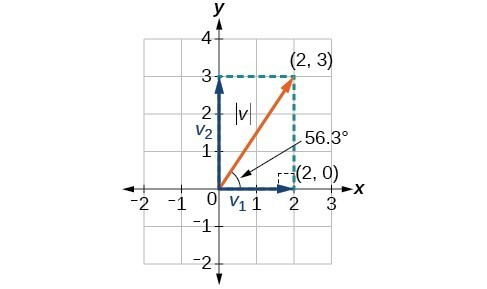Figure 12

Thus, the magnitude of $\boldsymbol{v}$ is $\sqrt{13}$ and the direction is ${56.3}^{\circ }$ off the horizontal.

### Example 8: Finding the Components of the Vector

Find the components of the vector $\boldsymbol{v}$ with initial point $\left(3,2\right)$ and terminal point $\left(7,4\right)$.

## Finding the Unit Vector in the Direction of v

In addition to finding a vector’s components, it is also useful in solving problems to find a vector in the same direction as the given vector, but of magnitude 1. We call a vector with a magnitude of 1 a unit vector. We can then preserve the direction of the original vector while simplifying calculations.

Unit vectors are defined in terms of components. The horizontal unit vector is written as $\boldsymbol{i}=\langle 1,0\rangle$ and is directed along the positive horizontal axis. The vertical unit vector is written as $\boldsymbol{j}=\langle 0,1\rangle$ and is directed along the positive vertical axis.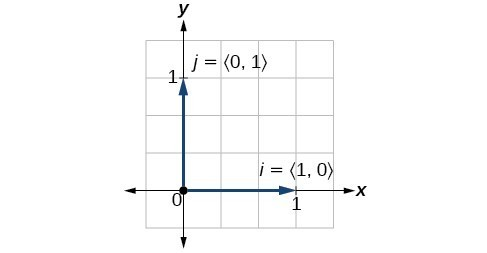Figure 14

### A General Note: The Unit Vectors

If $\boldsymbol{v}$ is a nonzero vector, then $\dfrac{\boldsymbol{v}}{|\boldsymbol{v}|}$ is a unit vector in the direction of $\boldsymbol{v}$. Any vector divided by its magnitude is a unit vector. Notice that magnitude is always a scalar, and dividing by a scalar is the same as multiplying by the reciprocal of the scalar.

### Example 9: Finding the Unit Vector in the Direction of v

Find a unit vector in the same direction as $\boldsymbol{v}=\langle -5,12\rangle$.

## Performing Operations with Vectors in Terms of i and j

So far, we have investigated the basics of vectors: magnitude and direction, vector addition and subtraction, scalar multiplication, the components of vectors, and the representation of vectors geometrically. Now that we are familiar with the general strategies used in working with vectors, we will represent vectors in rectangular coordinates in terms of i and j.

### A General Note: Vectors in the Rectangular Plane

Given a vector $v$ with initial point $P=\left({x}_{1},{y}_{1}\right)$ and terminal point $Q=\left({x}_{2},{y}_{2}\right)$, v is written as

$\boldsymbol{v}=\left({x}_{2}-{x}_{1}\right)\boldsymbol{i}+\left({y}_{1}-{y}_{2}\right)\boldsymbol{j}$

The position vector from $\left(0,0\right)$ to $\left(a,b\right)$, where $\left({x}_{2}-{x}_{1}\right)=a$ and $\left({y}_{2}-{y}_{1}\right)=b$, is written as v = ai + bj. This vector sum is called a linear combination of the vectors i and j.

The magnitude of v = ai + bj is given as $|\boldsymbol{v}|=\sqrt{{a}^{2}+{b}^{2}}$.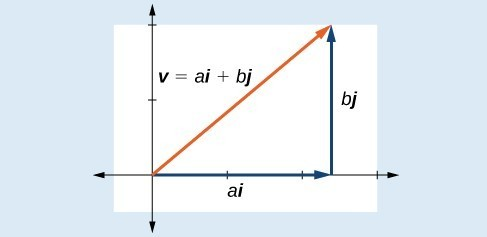Figure 16

### Example 10: Writing a Vector in Terms of i and j

Given a vector $\boldsymbol{v}$ with initial point $P=\left(2,-6\right)$ and terminal point $Q=\left(-6,6\right)$, write the vector in terms of $\boldsymbol{i}$ and $\boldsymbol{j}$.

### Example 11: Writing a Vector in Terms of i and j Using Initial and Terminal Points

Given initial point ${P}_{1}=\left(-1,3\right)$ and terminal point ${P}_{2}=\left(2,7\right)$, write the vector $\boldsymbol{v}$ in terms of $\boldsymbol{i}$ and $\boldsymbol{j}$.

### Try It

Write the vector $\boldsymbol{u}$ with initial point $P=\left(-1,6\right)$ and terminal point $Q=\left(7,-5\right)$ in terms of $\boldsymbol{i}$ and $\boldsymbol{j}$.

## Performing Operations on Vectors in Terms of i and j

When vectors are written in terms of i and j, we can carry out addition, subtraction, and scalar multiplication by performing operations on corresponding components.

### A General Note: Adding and Subtracting Vectors in Rectangular Coordinates

Given v = ai + bj and u = ci + dj, then

$\begin{gathered}\boldsymbol{v}+\boldsymbol{u}=\left(a+c\right)\boldsymbol{i}+\left(b+d\right)\boldsymbol{j}\\ \boldsymbol{v}-\boldsymbol{u}=\left(a-c\right)\boldsymbol{i}+\left(b-d\right)\boldsymbol{j}\end{gathered}$

### Example 12: Finding the Sum of the Vectors

Find the sum of ${\boldsymbol{v}}_{1}=2\boldsymbol{i} - 3\boldsymbol{j}$ and ${\boldsymbol{v}}_{2}=4\boldsymbol{i}+5\boldsymbol{j}$.

## Calculating the Component Form of a Vector: Direction

We have seen how to draw vectors according to their initial and terminal points and how to find the position vector. We have also examined notation for vectors drawn specifically in the Cartesian coordinate plane using $i\text{and}j$. For any of these vectors, we can calculate the magnitude. Now, we want to combine the key points, and look further at the ideas of magnitude and direction.

Calculating direction follows the same straightforward process we used for polar coordinates. We find the direction of the vector by finding the angle to the horizontal. We do this by using the basic trigonometric identities, but with |vreplacing r.

### A General Note: Vector Components in Terms of Magnitude and Direction

Given a position vector $\boldsymbol{v}=\langle x,y\rangle$ and a direction angle $\theta$,

\begin{align}&\cos \theta =\frac{x}{|\boldsymbol{v}|}&& \text{and}&& \sin \theta =\frac{y}{|\boldsymbol{v}|} \\ &x=|\boldsymbol{v}|\cos \theta &&&& y=|\boldsymbol{v}|\sin \theta \end{align}

Thus, $\boldsymbol{v}=x\boldsymbol{i}+y\boldsymbol{j}=|\boldsymbol{v}|\cos \theta \boldsymbol{i}+|v|\sin \theta \boldsymbol{j}$, and magnitude is expressed as $|\boldsymbol{v}|=\sqrt{{x}^{2}+{y}^{2}}$.

### Example 13: Writing a Vector in Terms of Magnitude and Direction

Write a vector with length 7 at an angle of 135° to the positive x-axis in terms of magnitude and direction.

### Try It

A vector travels from the origin to the point $\left(3,5\right)$. Write the vector in terms of magnitude and direction.

## Resultant Forces

Many physics concepts can be represented by vectors. A vector that represents a pull or push of some type is called a force vector. If $F_1$ and $F_2$ are two forces acting on an object, the net effect is the same as if just the resultant force $F_1+F_2$ acted on the object. If the object is not moving, such as if you hand a picture on the wall, the vector sum of all forces is the zero vector. This is called static equilibrium. The engineering course called statics studies systems in which the zero vector always occurs.

### A General Note: How to find magnitude and direction of a resultant force

If $\boldsymbol{F}=a\boldsymbol{i}+b\boldsymbol{j}$ represents a resultant force, the following formulas can be used to find its magnitude and direction:

magnitude: $||\boldsymbol{F}||=\sqrt{a^{2}+b^{2}}$

direction: \begin{align}&\theta=\tan^{-1}\left(\frac{b}{a}\right)&&\text{ if the resultant vector is in quadrant II or III.}\\ &\theta =\tan^{-1}\left(\frac{b}{a}\right)+180^\circ && \text{ if the resultant vector is in quadrant I or IV.}\end{align}

### Example 14: Find the magnitude and direction of the resultant force

Find the magnitude and direction of the resultant force:
30 pounds at $27^\circ$
40 pounds at $136^\circ$

### Try It

Find the magnitude and direction of the resultant force. Use two decimal places.
7 pounds at $15^\circ$
13 pounds at $170^\circ$

## Finding the Dot Product of Two Vectors

As we discussed earlier in the section, scalar multiplication involves multiplying a vector by a scalar, and the result is a vector. As we have seen, multiplying a vector by a number is called scalar multiplication. If we multiply a vector by a vector, there are two possibilities: the dot product and the cross product. We will only examine the dot product here; you may encounter the cross product in more advanced mathematics courses.

The dot product of two vectors involves multiplying two vectors together, and the result is a scalar.

### A General Note: Dot Product

The dot product of two vectors $\boldsymbol{v}=\langle a,b\rangle$ and $\boldsymbol{v}=\langle c,d\rangle$ is the sum of the product of the horizontal components and the product of the vertical components.

$\boldsymbol{v}\cdot \boldsymbol{u}=ac+bd$

To find the angle between the two vectors, use the formula below.

$\cos \theta =\dfrac{\boldsymbol{v}}{|\boldsymbol{v}|}\cdot \dfrac{\boldsymbol{u}}{|\boldsymbol{u}|}$

### Example 15: Finding the Dot Product of Two Vectors

Find the dot product of $\boldsymbol{v}=\langle 5,12\rangle$ and $\boldsymbol{u}=\langle -3,4\rangle$.

### Example 16: Finding the Dot Product of Two Vectors and the Angle between Them

Find the dot product of v1 = 5i + 2j and v2 = 3i + 7j. Then, find the angle between the two vectors.

### Example 17: Finding the Angle between Two Vectors

Find the angle between $\boldsymbol{u}=\langle -3,4\rangle$ and $\boldsymbol{v}=\langle 5,12\rangle$.

### Example 18: Finding Ground Speed and Bearing Using Vectors

We now have the tools to solve the problem we introduced in the opening of the section.

An airplane is flying at an airspeed of 200 miles per hour headed on a SE bearing of 140°. A north wind (from north to south) is blowing at 16.2 miles per hour. What are the ground speed and actual bearing of the plane?Figure 19

## Key Concepts

• The position vector has its initial point at the origin.
• If the position vector is the same for two vectors, they are equal.
• Vectors are defined by their magnitude and direction.
• If two vectors have the same magnitude and direction, they are equal.
• Vector addition and subtraction result in a new vector found by adding or subtracting corresponding elements.
• Scalar multiplication is multiplying a vector by a constant. Only the magnitude changes; the direction stays the same.
• Vectors are comprised of two components: the horizontal component along the positive x-axis, and the vertical component along the positive y-axis.
• The unit vector in the same direction of any nonzero vector is found by dividing the vector by its magnitude.
• The magnitude of a vector in the rectangular coordinate system is $|\boldsymbol{v}|=\sqrt{{a}^{2}+{b}^{2}}$.
• In the rectangular coordinate system, unit vectors may be represented in terms of i and j where i represents the horizontal component and j represents the vertical component. Then, v = ai + bj is a scalar multiple of v by real numbers $a\text{ and }b$.
• Adding and subtracting vectors in terms of i and j consists of adding or subtracting corresponding coefficients of i and corresponding coefficients of j.
• A vector v = ai + bj is written in terms of magnitude and direction as $\boldsymbol{v}=|\boldsymbol{v}|\cos \theta \boldsymbol{i}+|\boldsymbol{v}|\sin \theta \boldsymbol{j}$.
• The dot product of two vectors is the product of the i terms plus the product of the j terms.
• We can use the dot product to find the angle between two vectors.
• Dot products are useful for many types of physics applications.

## Glossary

dot product
given two vectors, the sum of the product of the horizontal components and the product of the vertical components
initial point
the origin of a vector
magnitude
the length of a vector; may represent a quantity such as speed, and is calculated using the Pythagorean Theorem
resultant
a vector that results from addition or subtraction of two vectors, or from scalar multiplication
scalar
a quantity associated with magnitude but not direction; a constant
scalar multiplication
the product of a constant and each component of a vector
standard position
the placement of a vector with the initial point at $\left(0,0\right)$ and the terminal point $\left(a,b\right)$, represented by the change in the x-coordinates and the change in the y-coordinates of the original vector
terminal point
the end point of a vector, usually represented by an arrow indicating its direction
unit vector
a vector that begins at the origin and has magnitude of 1; the horizontal unit vector runs along the x-axis and is defined as ${v}_{1}=\langle 1,0\rangle$ the vertical unit vector runs along the y-axis and is defined as ${v}_{2}=\langle 0,1\rangle$.
vector
a quantity associated with both magnitude and direction, represented as a directed line segment with a starting point (initial point) and an end point (terminal point)
the sum of two vectors, found by adding corresponding components

## Section 6.6 Homework Exercises

1. What are the characteristics of the letters that are commonly used to represent vectors?

2. How is a vector more specific than a line segment?

3. What are i and j, and what do they represent?

4. What is component form?

5. When a unit vector is expressed as $\langle a,b\rangle$, which letter is the coefficient of the i and which the j?

6. Given a vector with initial point (5,2) and terminal point (−1,−3), find an equivalent vector whose initial point is (0,0). Write the vector in component form $\langle a,b\rangle$.

7. Given a vector with initial point (−4,2) and terminal point (3,−3), find an equivalent vector whose initial point is (0,0). Write the vector in component form $\langle a,b\rangle$.

8. Given a vector with initial point (7,−1) and terminal point (−1,−7), find an equivalent vector whose initial point is (0,0). Write the vector in component form $\langle a,b\rangle$.

For the following exercises, determine whether the two vectors u and v are equal, where u has an initial point $P_{1}$ and a terminal point $P_{2}$ and v has an initial point $P3$ and a terminal point $P4$.

9. $P_{1}=\left(5,1\right)\text{, }P_{2}=\left(3,−2\right),P_{3}=\left(−1,3\right)$, and $P_{4}=\left(9,−4\right)$

10. $P_{1}=\left(2,−3\right),P_{2}=\left(5,1\right),P_{3}=\left(6,−1\right)$, and $P_{4}=\left(9,3\right)$

11. $P_{1}=\left(−1,−1\right),P_{2}=\left(−4,5\right),P_{3}=\left(−10,6\right)$, and $P_{4}=\left(−13,12\right)$

12. $P_{1}=\left(3,7\right),P_{2}=\left(2,1\right),P_{3}=\left(1,2\right)$, and $P_{4}=\left(−1,−4\right)$

13. $P_{1}=\left(8,3\right),P_{2}=\left(6,5\right),P_{3}=\left(11,8\right)$, and $P_{4}=\left(9,10\right)$

14. Given initial point $P_{1}=\left(−3,1\right)$ and terminal point $P_{2}=\left(5,2\right)$, write the vector in terms of and j.

15. Given initial point $P_{1}=\left(6,0\right)$ and terminal point $P_{2}=\left(−1,−3\right)$, write the vector v in terms of i and j.

For the following exercises, use the vectors $\boldsymbol{u}=\boldsymbol{i}+5\boldsymbol{j}$, $\boldsymbol{v}=−2\boldsymbol{i}−3\boldsymbol{j}$, and $\boldsymbol{w}=4\boldsymbol{i}−\boldsymbol{j}$.

16. Find $u+\left(\boldsymbol{v}−\boldsymbol{w}\right)$

17. Find $4\boldsymbol{v}+2\boldsymbol{u}$

For the following exercises, use the given vectors to compute $\boldsymbol{u}+\boldsymbol{v}$, $\boldsymbol{u}−\boldsymbol{v}$, and $2\boldsymbol{u}−3\boldsymbol{v}$.

18. $\boldsymbol{u}=\langle 2,−3\rangle,\boldsymbol{v}=\langle 1,5\rangle$

19. $\boldsymbol{u}=\langle−3,4\rangle,\boldsymbol{v}=\langle−2,1\rangle$

20. Let $\boldsymbol{v}=−4\boldsymbol{i}+3\boldsymbol{j}$. Find a vector that is half the length and points in the same direction as v.

21. Let $\boldsymbol{v}=5\boldsymbol{i}+2\boldsymbol{j}$. Find a vector that is twice the length and points in the opposite direction as v.

For the following exercises, find a unit vector in the same direction as the given vector.

22. $\boldsymbol{a}=3\boldsymbol{i}+4\boldsymbol{j}$

23. $\boldsymbol{b}=−2\boldsymbol{i}+5\boldsymbol{j}$

24. $\boldsymbol{c}=10\boldsymbol{i}–\boldsymbol{j}$

25. $\boldsymbol{d}=–\frac{1}{3}\boldsymbol{i}+\frac{5}{2}\boldsymbol{j}$

26. $\boldsymbol{u}=100\boldsymbol{i}+200\boldsymbol{j}$

27. $\boldsymbol{u}=–14\boldsymbol{i}+2\boldsymbol{j}$

For the following exercises, find the magnitude and direction of the vector, $0\leq\theta<2\pi$.

28. $\langle 0,4\rangle$

29. $\langle 6,5\rangle$

30. $\langle 2,–5\rangle$

31. $\langle –4,–6\rangle$

32. Given $\boldsymbol{u}=3\boldsymbol{i}−4\boldsymbol{j}$ and $\boldsymbol{v}=−2\boldsymbol{i}+3\boldsymbol{j}$, calculate $\boldsymbol{u}\cdot \boldsymbol{v}$.

33. Given $\boldsymbol{u}=−\boldsymbol{i}−\boldsymbol{j}$ and $\boldsymbol{v}=\boldsymbol{i}+5\boldsymbol{j}$, calculate $\boldsymbol{u}\cdot \boldsymbol{v}$.

34. Given $\boldsymbol{u}=\langle−2,4\rangle$ and $\boldsymbol{v}=\langle−3,1\rangle$, calculate $\boldsymbol{u}\cdot \boldsymbol{v}$.

35. Given $\boldsymbol{u}=\langle−1,6\rangle$ and $\boldsymbol{v}=\langle 6,−1\rangle$, calculate $\boldsymbol{u}\cdot \boldsymbol{v}$.

For the following exercises, given v, draw v, 3v, and $\frac{1}{2}\boldsymbol{v}$.

36. $\langle 2,−1\rangle$

37. $\langle −1,4\rangle$

38. $\langle−3,−2\rangle$

For the following exercises, use the vectors shown to sketch $\boldsymbol{u}+\boldsymbol{v}$, $\boldsymbol{u}−\boldsymbol{v}$, and $2\boldsymbol{u}$.

39.40.41.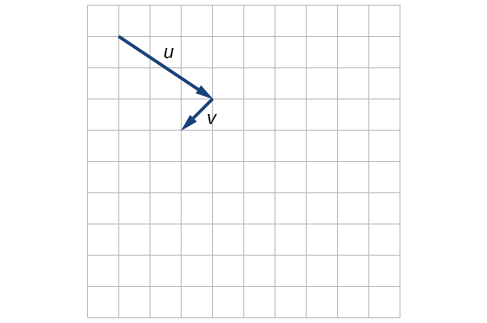For the following exercises, use the vectors shown to sketch $2\boldsymbol{u}+\boldsymbol{v}$.

42.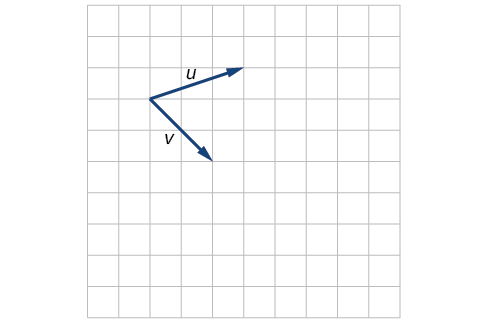43.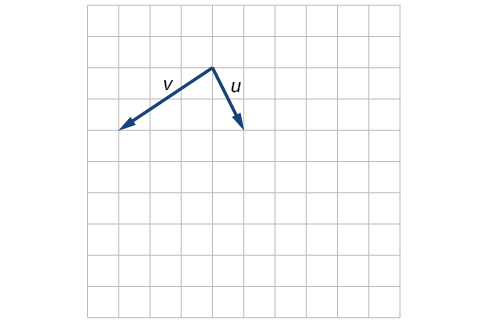For the following exercises, use the vectors shown to sketch $\boldsymbol{u}−3\boldsymbol{v}$.

44.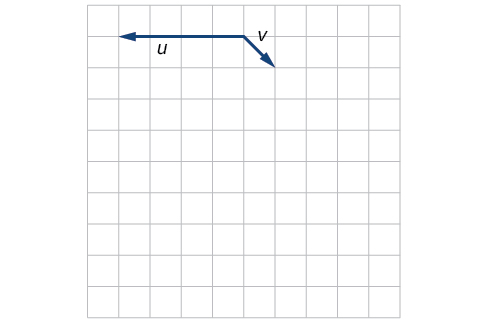45.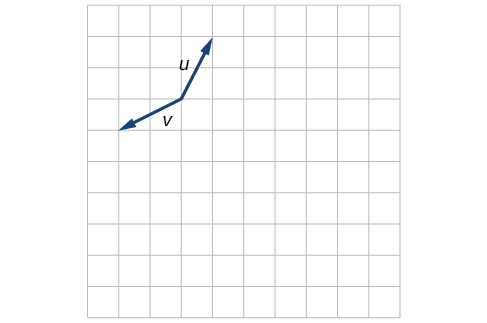For the following exercises, write the vector shown in component form.

46.47.48. Given initial point $P_{1}=\left(2,1\right)$ and terminal point $P_{2}=\left(−1,2\right)$,write the vector v in terms of i and j, then draw the vector on the graph.

49. Given initial point $P_{1}=\left(4,−1\right)$ and terminal point $P_{2}=\left(−3,2\right)$, write the vector v in terms of i and j. Draw the points and the vector on the graph.

50. Given initial point $P_{1}=\left(3,3\right)$ and terminal point $P_{2}=\left(−3,3\right)$, write the vector v in terms of i and j. Draw the points and the vector on the graph.

For the following exercises, use the given magnitude and direction in standard position, write the vector in component form.

51. $|\boldsymbol{v}|=6,\theta=45^{\circ}$

52. $|\boldsymbol{v}|=8,\theta=220^{\circ}$

53. $|\boldsymbol{v}|=2,\theta=300^{\circ}$

54. $|\boldsymbol{v}|=5,\theta=135^{\circ}$

55. Two forces of 10 pounds and 20 pounds act on an object at angles of 50 degrees and 160 degrees, respectively.  Find the magnitude and direction of the resultant force, rounded to two decimal places.

56. Two forces of 5 pounds and 15 pounds act on an object at angles of 40 degrees and 155 degrees, respectively.  Find the magnitude and direction of the resultant force, rounded to two decimal places.

57. Two forces of 3 newtons and 7 newtons act on an object at angles of 10 degrees and 295 degrees, respectively.  Find the magnitude and direction of the resultant force, rounded to two decimal places.

58. Two forces of 12 newtons and 19 newtons act on an object at angles of 15 degrees and 250 degrees, respectively.  Find the magnitude and direction of the resultant force, rounded to two decimal places.

59. A 60-pound box is resting on a ramp that is inclined 12°. Rounding to the nearest tenth,

Find the magnitude of the normal (perpendicular) component of the force.
Find the magnitude of the component of the force that is parallel to the ramp.

60. A 25-pound box is resting on a ramp that is inclined 8°. Rounding to the nearest tenth,

Find the magnitude of the normal (perpendicular) component of the force.
Find the magnitude of the component of the force that is parallel to the ramp.

61. Find the magnitude of the horizontal and vertical components of a vector with magnitude 8 pounds pointed in a direction of $27^{\circ}$ above the horizontal. Round to the nearest hundredth.

62. Find the magnitude of the horizontal and vertical components of the vector with magnitude 4 pounds pointed in a direction of $127^{\circ}$ above the horizontal. Round to the nearest hundredth.

63. Find the magnitude of the horizontal and vertical components of the vector with magnitude 5 pounds pointed in a direction of $55^{\circ}$ above the horizontal. Round to the nearest hundredth.

64. Find the magnitude of the horizontal and vertical components of the vector with magnitude 1 pound pointed in a direction of $8^{\circ}$ above the horizontal. Round to the nearest hundredth.

65. A woman leaves home and walks 3 miles west, then 2 miles southwest. How far from home is she, and in what direction must she walk to head directly home?

66. A boat leaves the marina and sails 6 miles north, then 2 miles northeast. How far from the marina is the boat, and in what direction must it sail to head directly back to the marina?

67. A man starts walking from home and walks 4 miles east, 2 miles southeast, 5 miles south, 4 miles southwest, and 2 miles east. How far has he walked? If he walked straight home, how far would he have to walk?

68. A woman starts walking from home and walks 4 miles east, 7 miles southeast, 6 miles south, 5 miles southwest, and 3 miles east. How far has she walked? If she walked straight home, how far would she have to walk?

69. A man starts walking from home and walks 3 miles at $20^{\circ}$ north of west, then 5 miles at $10^{\circ}$ west of south, then 4 miles at $15^{\circ}$ north of east. If he walked straight home, how far would he have to the walk, and in what direction?

70. A woman starts walking from home and walks 6 miles at $40^{\circ}$ north of east, then 2 miles at $15^{\circ}$ east of south, then 5 miles at $30^{\circ}$ south of west. If she walked straight home, how far would she have to walk, and in what direction?

71. An airplane is heading north at an airspeed of 600 km/hr, but there is a wind blowing from the southwest at 80 km/hr. How many degrees off course will the plane end up flying, and what is the plane’s speed relative to the ground?

72. An airplane is heading north at an airspeed of 500 km/hr, but there is a wind blowing from the northwest at 50 km/hr. How many degrees off course will the plane end up flying, and what is the plane’s speed relative to the ground?

73. An airplane needs to head due north, but there is a wind blowing from the southwest at 60 km/hr. The plane flies with an airspeed of 550 km/hr. To end up flying due north, how many degrees west of north will the pilot need to fly the plane?

74. An airplane needs to head due north, but there is a wind blowing from the northwest at 80 km/hr. The plane flies with an airspeed of 500 km/hr. To end up flying due north, how many degrees west of north will the pilot need to fly the plane?

75. As part of a video game, the point (5,7) is rotated counterclockwise about the origin through an angle of $35^{\circ}$. Find the new coordinates of this point.

76. As part of a video game, the point (7,3) is rotated counterclockwise about the origin through an angle of $40^{\circ}$. Find the new coordinates of this point.

77. Two children are throwing a ball back and forth straight across the back seat of a car. The ball is being thrown 10 mph relative to the car, and the car is traveling 25 mph down the road. If one child doesn’t catch the ball, and it flies out the window, in what direction does the ball fly (ignoring wind resistance)?

78. Two children are throwing a ball back and forth straight across the back seat of a car. The ball is being thrown 8 mph relative to the car, and the car is traveling 45 mph down the road. If one child doesn’t catch the ball, and it flies out the window, in what direction does the ball fly (ignoring wind resistance)?

79. A 50-pound object rests on a ramp that is inclined 19°. Find the magnitude of the components of the force parallel to and perpendicular to (normal) the ramp to the nearest tenth of a pound.

80. Suppose a body has a force of 10 pounds acting on it to the right, 25 pounds acting on it upward, and 5 pounds acting on it 45° from the horizontal. What single force is the resultant force acting on the body?

81. Suppose a body has a force of 10 pounds acting on it to the right, 25 pounds acting on it ─135° from the horizontal, and 5 pounds acting on it directed 150° from the horizontal. What single force is the resultant force acting on the body?

82. The condition of equilibrium is when the sum of the forces acting on a body is the zero vector. Suppose a body has a force of 2 pounds acting on it to the right, 5 pounds acting on it upward, and 3 pounds acting on it 45° from the horizontal. What single force is needed to produce a state of equilibrium on the body?

83. Suppose a body has a force of 3 pounds acting on it to the left, 4 pounds acting on it upward, and 2 pounds acting on it $30^{\circ}$ from the horizontal. What single force is needed to produce a state of equilibrium on the body? Draw the vector.